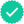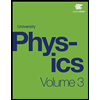Question

A light-year is the distance that light can travel in one year. Similarly, we can define a light-second, light-day, etc. as the distance that light can travel in other time intervals. Calculate the distance represented by each of the following: (Assume that the speed of light is 3 x 108 m/s)
A. 4 light-seconds.
B. [3pt] 4 light-minutes.
C. 4 light-days.
D. 4 light-days, but this time answer in miles (enter just the number with no units)

Expert SolutionStudents who’ve seen this question also like:Your question is solved by a Subject Matter ExpertWant to see this answer and more?
Experts are waiting 24/7 to provide step-by-step solutions in as fast as 30 minutes!*
*Response times may vary by subject and question complexity. Median response time is 34 minutes for paid subscribers and may be longer for promotional offers.8+ million solutions